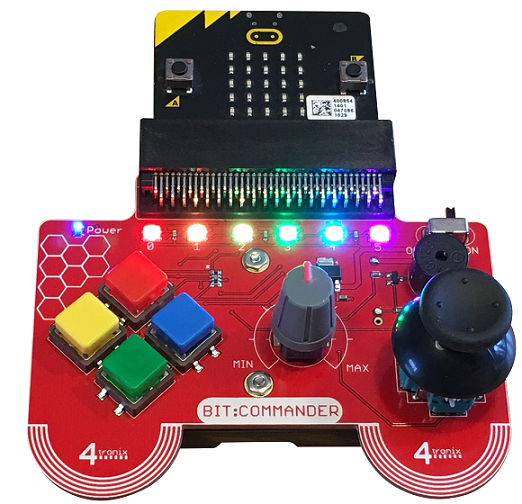# BBC micro:bit Bit:Commander Lights Out

## Introduction

When I looked through the programs I had, thinking of which ones could do with some tidier input, the Lights Out puzzle game was one that I thought of quickly. See the other page for a brief explanation of the solution and a link to follow for more information.```from microbit import *
import random

x = 2
y = 2
tick = -1

grid = [
[0,0,0,0,0],
[0,0,0,0,0],
[0,0,0,0,0],
[0,0,0,0,0],
[0,0,0,0,0]
]

# A 'move' in Lights Out
def Toggle(tx, ty):
grid[tx][ty] ^= 1
if tx>0:
grid[tx-1][ty] ^= 1
if tx<4:
grid[tx+1][ty] ^= 1
if ty>0:
grid[tx][ty-1] ^= 1
if ty<4:
grid[tx][ty+1] ^= 1

def RandomGrid():
for r in range(0,50):
cx = random.randint(0,4)
cy = random.randint(0,4)
Toggle(cx, cy)

def DrawGame(t):
img = Image('00000:'*5)
for cy in range(0,5):
for cx in range(0,5):
img.set_pixel(cx, cy, grid[cx][cy]*5)
img.set_pixel(x, y, (t % 2)*9)
return img

def CheckWin():
tot = 0
for cy in range(0,5):
for cx in range(0,5):
tot += grid[cx][cy]
if tot == 0:
return True
else:
return False

# set the board up for a game
RandomGrid()

while True:
tick +=1
if tick==2:
tick = 0
# check for movement
if dx > 850:
x += 1
sleep(200)
if dx < 150:
x -= 1
sleep(200)
if dy > 850:
y -= 1
sleep(200)
if dy < 150:
y += 1
sleep(200)
# keep on grid
x = max(0, min(x, 4))
y = max(0, min(y, 4))
# check for button press
Toggle(x, y)
sleep(200)
# update screen
i = DrawGame(tick)
display.show(i)
if CheckWin():
sleep(1000)
for w in range(0,6):
display.show(Image.HAPPY)
sleep(500)
display.clear()
sleep(500)
RandomGrid()
x = 2
y = 2
i = DrawGame(tick)
sleep(50)
```